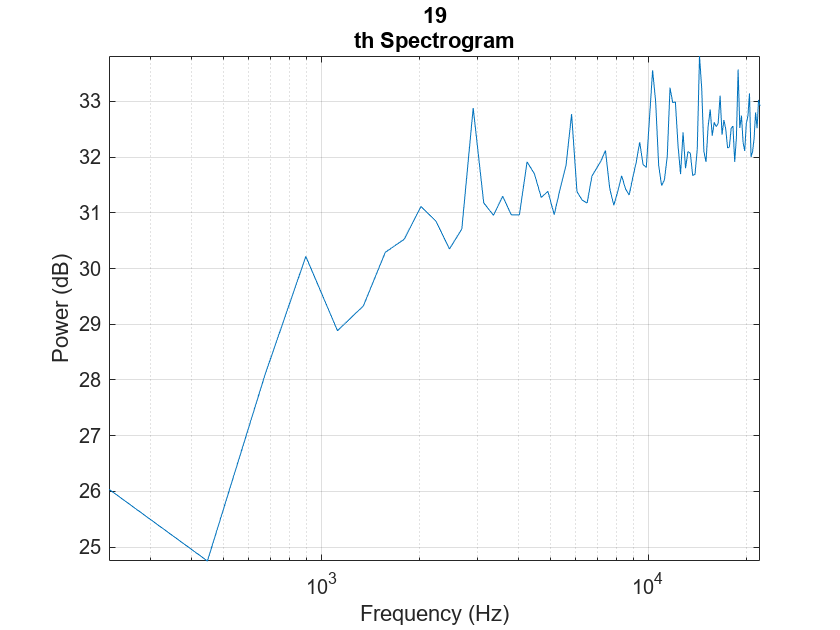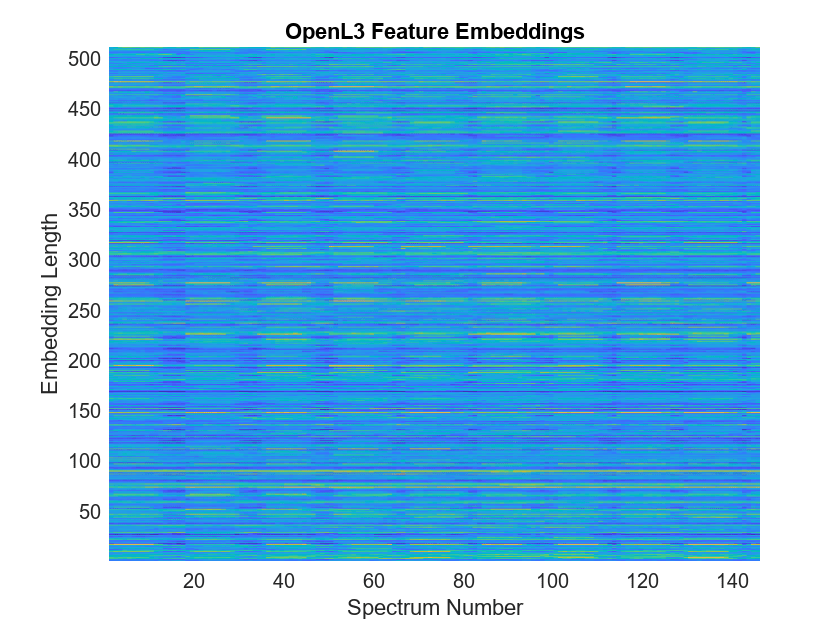# openl3Preprocess

Preprocess audio for OpenL3 feature extraction

Since R2021a

## Syntax

``features = openl3Preprocess(audioIn,fs)``
``features = openl3Preprocess(audioIn,fs,Name,Value)``

## Description

example

````features = openl3Preprocess(audioIn,fs)` generates spectrograms from `audioIn` that can be fed to the OpenL3 pretrained network.```
````features = openl3Preprocess(audioIn,fs,Name,Value)` specifies options using one or more `Name,Value` arguments. For example, `features = openl3Preprocess(audioIn,fs,'OverlapPercentage',75)` applies a 75% overlap between consecutive frames used to generate the spectrograms.```

## Examples

collapse all

Type `openl3` at the Command Window. If the Audio Toolbox model for OpenL3 is not installed, the function provides a link to the location of the network weights. To download the model, click the link. Unzip the file to a location on the MATLAB path.

```downloadFolder = fullfile(tempdir,'OpenL3Download'); loc = websave(downloadFolder,'https://ssd.mathworks.com/supportfiles/audio/openl3.zip'); OpenL3Location = tempdir; unzip(loc,OpenL3Location) addpath(fullfile(OpenL3Location,'openl3'))```

Check that the installation is successful by typing `openl3` at the Command Window. If the network is installed, then the function returns a `DAGNetwork` (Deep Learning Toolbox) object.

`openl3`
```ans = DAGNetwork with properties: Layers: [30×1 nnet.cnn.layer.Layer] Connections: [29×2 table] InputNames: {'in'} OutputNames: {'out'} ```

Use `openl3Preprocess` to extract embeddings from an audio signal.

`[audioIn,fs] = audioread('Counting-16-44p1-mono-15secs.wav');`

To extract spectrograms from the audio, call the `openl3Preprocess` function with the audio and sample rate. Use 50% overlap and set the spectrum type to linear. The `openl3Preprocess` function returns an array of 30 spectrograms produced using an FFT length of 512.

```features = openl3Preprocess(audioIn,fs,'OverlapPercentage',50,'SpectrumType','linear'); [posFFTbinsOvLap50,numHopsOvLap50,~,numSpectOvLap50] = size(features)```
```posFFTbinsOvLap50 = 257 ```
```numHopsOvLap50 = 197 ```
```numSpectOvLap50 = 30 ```

Call `openl3Preprocess` again, this time using the default overlap of 90%. The `openl3Preprocess` function now returns an array of 146 spectrograms.

```features = openl3Preprocess(audioIn,fs,'SpectrumType','linear'); [posFFTbinsOvLap90,numHopsOvLap90,~,numSpectOvLap90] = size(features)```
```posFFTbinsOvLap90 = 257 ```
```numHopsOvLap90 = 197 ```
```numSpectOvLap90 = 146 ```

Visualize one of the spectrograms at random.

```randSpect = randi(numSpectOvLap90); viewRandSpect = features(:,:,:,randSpect); N = size(viewRandSpect,2); binsToHz = (0:N-1)*fs/N; nyquistBin = round(N/2); semilogx(binsToHz(1:nyquistBin),mag2db(abs(viewRandSpect(1:nyquistBin)))) xlabel('Frequency (Hz)') ylabel('Power (dB)'); title([num2str(randSpect),'th Spectrogram']) axis tight grid on```Create an OpenL3 network (this requires Deep Learning Toolbox) using the same `'SpectrumType'`.

`net = openl3('SpectrumType','linear');`

Extract and visualize the audio embeddings.

```embeddings = predict(net,features); surf(embeddings,'EdgeColor','none') view([90,-90]) axis([1 numSpectOvLap90 1 numSpectOvLap90]) xlabel('Embedding Length') ylabel('Spectrum Number') title('OpenL3 Feature Embeddings') axis tight```## Input Arguments

collapse all

Input signal, specified as a column vector or matrix. If you specify a matrix, `openl3Preprocess` treats the columns of the matrix as individual audio channels.

Data Types: `single` | `double`

Sample rate of the input signal in Hz, specified as a positive scalar.

Data Types: `single` | `double`

### Name-Value Arguments

Specify optional pairs of arguments as `Name1=Value1,...,NameN=ValueN`, where `Name` is the argument name and `Value` is the corresponding value. Name-value arguments must appear after other arguments, but the order of the pairs does not matter.

Before R2021a, use commas to separate each name and value, and enclose `Name` in quotes.

Example: `openl3Preprocess(audioIn,fs,'SpectrumType','mel256')`

Percentage overlap between consecutive spectrograms, specified as a scalar in the range [0,100).

Data Types: `single` | `double`

Spectrum type generated from audio and used as input to the neural network, specified as one of these:

• `'mel128'` –– Generates mel spectrograms using 128 mel bands.

• `'mel256'` –– Generates mel spectrograms using 256 mel bands.

• `'linear'` –– Generates positive one-sided spectrograms using an FFT length of 512.

Data Types: `char` | `string`

## Output Arguments

collapse all

Spectrograms generated from `audioIn`, returned as an N-by-M-by-1-by-K array.

When you specify `'SpectrumType'` as one of these:

• `'mel128'` –– The dimensions are `128`-by-`199`-by-`1`-by-K, where `128` is the number of mel bands and `199` is the number of time hops.

• `'mel256'` –– The dimensions are `256`-by-`199`-by-`1`-by-K, where `256` is the number of mel bands and `199` is the number of time hops.

• `'linear'` –– The dimensions are `257`-by-`197`-by-`1`-by-K, where `257` is the positive one-sided FFT length and `197` is the number of time hops.

• K represents the number of spectrograms and depends on the length of `audioIn`, the number of channels in `audioIn`, as well as `OverlapPercentage`.

Data Types: `single`

 Cramer, Jason, et al. "Look, Listen, and Learn More: Design Choices for Deep Audio Embeddings." In ICASSP 2019 IEEE International Conference on Acoustics, Speech and Signal Processing (ICASSP), IEEE, 2019, pp. 3852-56. DOI.org (Crossref), doi:/10.1109/ICASSP.2019.8682475.